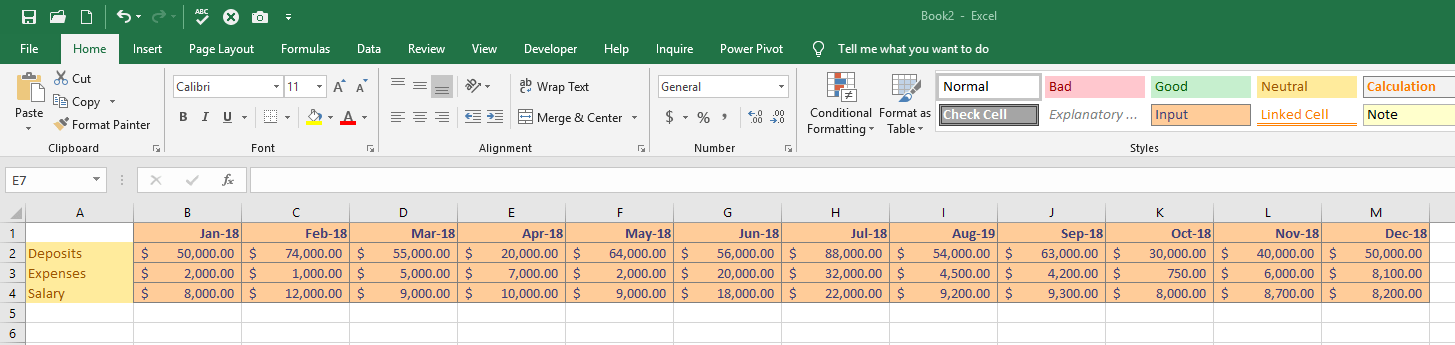# Excel – VLOOKUP and OFFSET with Profit and Expense

VLOOKUP and OFFSET can be combined in Microsoft Excel to produce quick and easy to read charts for analyzing your net profits, expenses, and total profit/loss. We’ll cover the basics of these formulas to demonstrate monthly p/l total calculations, along with using Data Validation to populate a drop-down list. Also, I’ll be showing a straightforward way to alter the original formula by using the SUM function and optional OFFSET function arguments (Height and Width) so that we can calculate an aggregate of our p/l factors through year end.

To start, put together the following grid in your Excel workbook. This represents Deposits, Expenses, and Salary costs for the entire year. In a real-world scenario, you’ll likely have more income-and-expense inputs, but for demo purposes…## VLOOKUP and Data Validation Table

In this section, we’ll setup the table to use for our Microsoft Excel VLOOKUP function. This table is also going to define the list of months that we’ll make available in a drop-down list with the use of the Data Validation > Allow > List.

We’ll create the table on another tab, and because of that, it’s essential to define Named Ranges for the columns of the table or else their data will be unavailable as references for use in the VLOOKUP and Data Validation features on the main worksheet.

As an aside, in case you’re not familiar with this feature: the Microsoft Excel Name Manager button lets you easily view and edit all of the Named Ranges, Tables, and other named objects in your workbook.## OFFSET and VLOOKUP Functions

Here we’ll look at how to combine the OFFSET and VLOOKUP functions together into a formula for updating the total net monthly profit-and-loss, and we’ll drive input into those functions through a drop-down list that’s powered by the Data Validation > Allow > List functionality in Microsoft Excel.

In advance, here’s the function I’ll use:

`=OFFSET(\$A2,0,VLOOKUP(\$B\$8,MonthTable,2,FALSE))`

And, here’s the table that will utilize that function as well as the Data Validation List:• The first argument to OFFSET is the reference column…the starting point.
• The second argument is the number of rows we want to offset. It’s set to zero because we don’t want to move up/down any number of rows.
• The third argument is the number of columns we want to offset. That’s going to be our MonthTable, second column, where the first column matches the month that the user selects in B8.

If you need further explanation, check out the Microsoft Excel in-product Help functionality…second to none.

Here we go:

Now you can add any charts that you like. I added a bar chart to show the comparison of our three categories against/versus the other. If you’ve followed along correctly, you’ll see the chart updates as you change the selected month.

# Advanced use of the Excel OFFSET Function – Height and Width

When you read through the documentation of the OFFSET function, you’ll find that there are arguments for Height and Width. This means that, as you shift between rows and columns you can select multiple cells offset from the reference/starting cell.

In my spreadsheet, I wanted to give users the ability to run the same calculation that’s been demonstrated, but instead of running it for the selected month only, they can now capture the values from the selected month through the end of the year. That formula looks as follows:

`=SUM(OFFSET(\$A2,0,VLOOKUP(\$B\$8,MonthTable,2,FALSE),1,13-VLOOKUP(\$B\$8,MonthTable,2,FALSE)))`

You can see that we’ve added the Microsoft Excel SUM function, and we’ve also implemented the Height and Width arguments.

• Height is “1” because we’re still only interested in a single row per output cell.
• Width is more interesting…it is a calculation.

From January, we’d want all 12 months (13 – 1).

From March, we’d want 10 months of calculations…(13 – 3).

…and so on. Go ahead and substitute in the new function above with the previous single-month formula and validate the results. Everything should work as expected.

Thanks for reading, and if you’d like to see another example of using VLOOKUP for powerful sales insights, check out my post on VLOOKUP and INDIRECT.